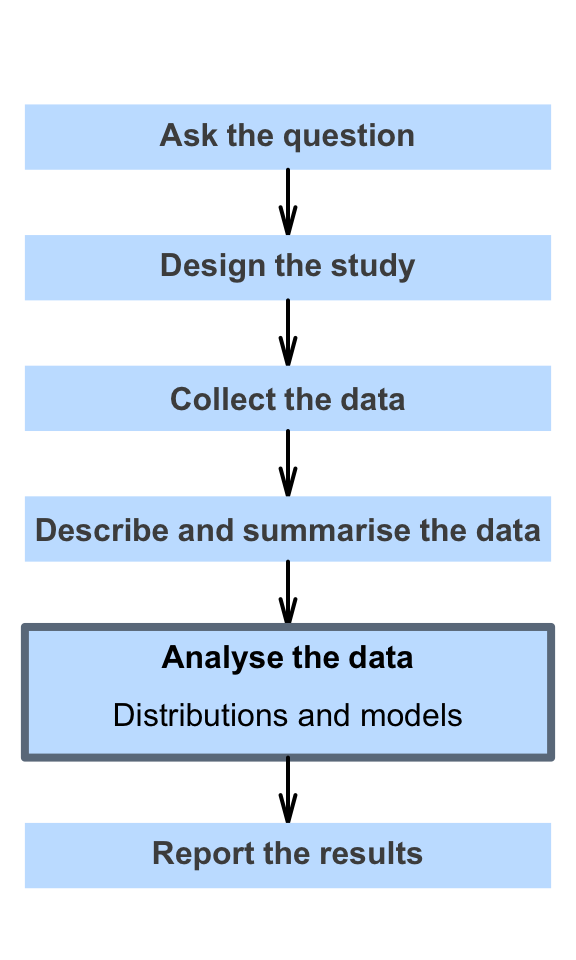# 17 Distributions and models

So far, you have learnt to ask a RQ, identify different ways of obtaining data, design the study, collect the data describe the data, summarise data graphically and numerically, and understand the decision-making process.

In this chapter, you will learn about distributions and models to describe the distribution of populations and samples. You will learn to:

• describe distributions.
• describe populations using normal distributions.
• use $$z$$-scores to compute probabilities related to normal distributions.
• use $$z$$-scores to ‘work backwards’ from probabilities for normal distributions.